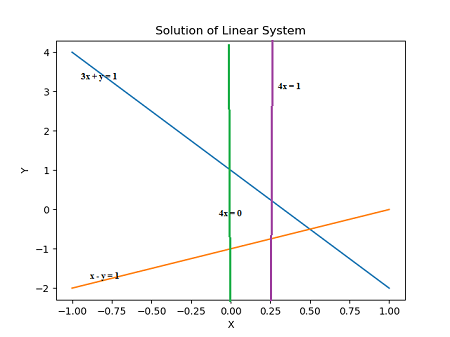# Determine whether each system is over determined or underdetermined; then solve each system; and...

## Question:

Determine whether each system is over determined or underdetermined; then solve each system; and confirm your answer by sketching the corresponding lines from the system.

{eq}(a) \ 3x + y = 1 \\ \ \ \ \ \ x - y = 1 \\ \ \ \ \ \ 4x = 1 \\ (b) \ 3x + y = 1 \\ \ \ \ \ \ x - y = 1 \\ \ \ \ \ \ 4x = 0 {/eq}

## Systems of Equations

The question gives us two linear systems of equations. We are to determine whether the systems are over determined or under determined. The concepts are important to consider when solving the systems of equations for a solution. Linear systems of equations have many important applications such as being used to design circuits in the area of Electrical Engineering.

(a) The first system of equations has two variables x and y while it has three equations. Therefore the system of equations is over determined.

The system of equations is inconsistent (no solution). Solving the third equation in the system gives us

{eq}\displaystyle 4x=1 \implies x = \frac 14. {/eq}

Substituting this value of x in the first equation gives us

{eq}\displaystyle \frac 34+y=1 \implies y=\frac 14. {/eq}

These values of x and y DO NOT satisfy the second equation since

{eq}\displaystyle \frac 14 - \frac 14 = 1 \implies 0 = 1 \; {\rm (CONTRADICTION)}. {/eq}

(b) The second system of equations has two variables x and y while it has three equations. Therefore the system of equations is over determined.

The system of equations is inconsistent (no solution). Solving the third equation in the system gives us

{eq}\displaystyle 4x=0 \implies x = 0. {/eq}

Substituting this value of x in the first equation gives us

{eq}\displaystyle 3(0)+y=1 \implies y=1. {/eq}

These values of x and y DO NOT satisfy the second equation since

{eq}\displaystyle 0-1=1 \implies -1 = 1 \; {\rm (CONTRADICTION)}. {/eq}

The sketch below shows that the three lines in either parts (a) or (b) DO NOT intersect in one point thereby making the system inconsistent. The graph was sketched using the PYTHON programming language package/module MATPLOTLIB: